# A TensorFlow Glossary/Cheat Sheet

There’s already a ton of great TensorFlow tutorials, Jupyter notebooks, and MOOCs out there. As someone who’s worked through quite a few this year, one stumbling block was that many tutorials would use terms I wasn’t familiar with. In addition, many of the tutorials use different levels of the TensorFlow API, or different high-level wrappers, adding to my confusion. I thought I’d write a glossary of terms I’ve encountered frequently to help out.

I am not a part of the TF team, and I’m still a student of TF and ML, so some of the below may be inaccurate, please add corrections to the comments.

I’ve also put the general ML concepts at the bottom, assuming many people trying to use TF already have some basic familiarity with ML. If not, the Coursera class is probably the best high-level intro. Finally, this is just a quick summary, most of these topics have more complete explanations on Wikipedia, research papers, online classes, etc.

Before I dive in, I’ll rehash some of those links to TF/ML tutorials:

• The official TF getting started and tutorials are of course on tensorflow.org
• Awesome Tensorflow is a huge compilation of TF tutorials
• The Keras examples are really good examples of popular architecture implementations
• Coursera has a world-famous ML classs and has now added a Deep Learning class. The full Stanford lectures that goes deeper into the math for the ML class is also on ITunes U.
• Udacity has a Deep Learning class and self-driving car class based on TensorFlow
• The Google Cloud ML sample repo has several tutorials, including tutorials that present the same problem solved with different levels of the API, which is a useful point of comparison.

Ok, onto the list, some of these definitions use vocabulary defined later:

• Acronyms: TF = TensorFlow. ML = Machine Learning

# TensorFlow API Levels

• Low-level API: TF code that makes TF Graphs of TF Ops. You can use this API for purposes other than ML as evidenced by the Mandelbrot tutorial. If you are more interested in solving an ML problem than creating new ML approaches, the higher level APIs are a better place to start, though understanding this level is also extremely helpful.
• Estimator API- A higher level API, and the most officially blessed one since it’s in the TF Github repo. It offers “Estimators” which are common ML models. It’s loosely based on the scikitlearn (sklearn) Python ML API. Very confusing point: at some point this has been referred to as the “tf learn” API because the code was in the `tf.contrib.learn` package in the TF repo, but it has no relation to the tflearn project mentioned below. You can also use the low-level API to implement your own Estimators. Implementing LinearClassifier yourself with the low-level API is a really good learning exercise.
• Experiment API- An official TensorFlow API designed to run long-running ML experiments. If training a model takes many hours or days, you want some standard ways of tracking metrics like accuracy, loss, a method to saving checkpoints of your models, etc. If you are using Google Cloud ML Engine to run TensorFlow, this is the best supported approach, and I’d highly recommend read this tutorial that uses it by Lak as well as check out the Cloud ML samples repo. Finally, since this package is in contrib, it’s an unstable API subject to major changes.
• tflearn — Another high level at tflearn.org TF API made independently of the TF team. Not related to the Estimator API, officially part of TF, or produced by Google. I don’t know much about this API
• Keras — Another high level API and probably the most popular. Predates TF but supports TF as one possible backend. Also loosely modeled after sklearn. This is becoming a more official entry point to TensorFlow since Google hired its creator.
• Layers — Core Tensorfow and Keras both offer layers, which are convienence functions for building a layer of a neural net, such as a convolutional layer. These are generally between the high level APIs and low-level APIs, because you are directly adding operations to the graph but using common patterns.
• tfslim — Yet another high level API like Keras, it’s in the official repo but not very popular
• Theano/PyTorch/Caffe — Popular deep learning frameworks that compete with TF.

# Core TensorFlow Concepts

• Tensor — A multi-dimensional matrix and fundamental part of the low-level API. To mathematicians this can be something more nuanced, but in TF it’s always just a multi-dimensional matrix.
• Graph/Ops/Session /Node— Often with the TF low-level API it seems like you are writing Python, but really you are just building a Graph of Ops (operations) that you will run later. The Session is how you run the Graph and maintains the state. A Node is a piece of the graph created by the Ops. One major point of confusion with TensorFlow is that often the code you write runs once you run the graph, not once you create the operation.
• Dense Tensor — A tensor whose values will typically be in a continuous range, meaning a single real valued features suffices
• Sparse Tensor — Actually a group of tensors that make it easier to represent spread-out or categorical values
• Feature columns — Often given a set of input data, you will want to combine or modify input features before you feed them into your network. Generally you need to map categorical features or string features to numerical values of some sort (real values or multi-dimensional tensors). This first level is called the feature columns.
• Feature crosses — A combination of two features, which is useful when the relationship between two features is important. See the Large Scale Linear Model tutorial.
• Queues — Tensorflow has a concept of queues. This is useful for reading in input features, where the inputs are too big to read into memory, so instead a worker loads the inputs into a queue and another operation pulls it off the queue for processing.

# Core Categorical Feature Concepts

Usually handling real-valued input functions in TF is significantly simpler than categorical features. We also usually treat free-form input like text as a categorical feature (for example, English input is categorical, with the possible values being the English language).

One temptation is just to map words to numerical values, perhaps with a hash function or just ordering from 0 to N, where N is the number of features. There is a problem though. Given 3 categories, red, green, and blue, if we map red to 0, green to 1, and blue to 2, we are making green and blue “closer” in value to the network than red and blue, even if we didn’t mean to. There are a variety of tools to deal with that.

• Vocabulary — The set of possible values for a categorical feature. It’s used to train the embeddings or create the hash buckets for categorical features.
• Hash buckets — The simplest way to map categorical features is just to hash their values, possibly into a set of buckets, whose number is determined or a hyperparameter.
• One hot encoding — Given a categorical input feature, if we simply map the possible values to numbers (say red->1, blue->2, green->3) we are putting red and blue closer in meaning (2–1=1), even if they aren’t any closer in meaning than red and green (3–1=2). Instead, a one hot encoding feature column would create 3 separate nodes for red, blue, and green, and have each one except the actual value be 0, and the actual value 1. This way red is no closer to blue than green.
• Softmax — Like a sigmoid function, but for multiple input values, it maps a set of numerical values to a set of probabilities that sum to 1. which is supposed to represent the probability of the input example being a given calss. A very common pattern is for the final layer of the network to have the same number of nodes as the number of possible classes, and then run the softmax function on the logits to generate probabilities for each of those classes.
• Logits- The very big or very small numbers that get mapped to probabilities. Techncially, logit is the inverse of the logistic (softmax) function, but in practice logits is used to refer to the value that would be obtained by running the logit function on the probabilities.
• Word embeddings-Technically any sort of numbers generated from words, but in a TF context word embeddings usually refers to creating vectors from categorical features and training those vectors. See the word2vec tutorial here. It provides more semantics than just a one-hot encoding since similar input features will have more similar values. Instead of a node for every possible value of the category, you use a fixed size vector (depth). The vectors are trained by the network, in the word2vec example, it’s used based on proximity in text, so that similar vector values are in fact closer in meaning. One interesting side-effect of this approach is that you can do vector math on the embeddings (e.g. king-queen = man).

# Popular Architectures

• Linear Classifier — Simple architecture that takes input features and combines them with weights and biases to predict an output value. One of the built in Esimators.
• DNNClassifier — Deep neural net classifiers. Involved intermediate layers of nodes that represent “hidden features” and activation functions to represent non-linearity. One of the built in Estimators.
• Wide and Deep — An architecture popularized by a Google paper that combines linear classifiers with deep neural net classifiers. The intuition is that the “wide” linear parts represent memorizing specific examples and the “deep” parts represent understanding high level features. For example, many parts of English grammar have rules based on parts-of-speech (learned by the deep part), but many common examples that break those (learned by the wide part). One of the built-in Estimators. See this tutorial.
• ConvNets — Convolutional neural nets. Popular architecture for image classification that uses grids that run across the input image to produce hidden layers. Examples include LeNet, Nvidia, ImageNet
• Transfer Learning — Models that use existing trained models as starting points and add additional layers for the specific use case. The intuition being the highly trained existing models know many general features that are a good starting point for training a small network on specific examples. The TensorFlow for Poets tutorial is a good example of doing this for image recognition.
• RNN — Recurrent neural nets, an architecture designed for handling a sequence of inputs that have “memory” of the sequence. LSTMs are a fancy version of RNNs. Very popular for Natural Language Processing (NLP) use cases
• GAN — General adversarial neural network, one model creates fake examples, and another model is served both fake example and real examples and is asked to distinguish. Popular approach to using ML to generate new data.

# Deployment Considerations

• GPU/TPU — Graphic processor units/Tensorflow processing units. GPUs are chips made for gaming cards optimized for matrix math. They are more or less essential for deep learning projects as they greatly speed up training. See this HN discussion on buying your own, or rent them on AWS or Google Cloud. TPUs were announced by Google as chips even more specialized for deep learning and will eventually be available on Google Cloud.
• Monitors/SessionRunHooks — Typically while training your model, you’ll want to keep an eye on your metrics like loss and accuracy. You can do this with many of the high level APIs by attaching Monitor. SessionRunHooks are the replacement for Monitors that have fewer problems by avoiding long running threads.
• TensorBoard — A GUI that comes with TensorFlow to show many of the relevant metrics during training
• Apache Spark/Apache Beam/Apache Hadoop — Popular Big Data Frameworks that are often used to preprocess features
• Apache Airflow — An Airbnb project that makes orchestrating all the monitoring/logging/preprocessing/model evaluation easier.
• Model staleness — Live models can drift in performance over time because the input data is outdated, so often in deployment, there are techniques to replace existing models with newer models, or the same model trained on newer data
• Google Cloud ML Engine — A managed Tensorflow Environment that provides training and prediction on a distributed cluster

# General ML Concepts

• Features — The input data used by the ML model
• Feature engineering — Transforming input features to be more useful for the models. Often includes things like mapping categories to buckets, regularizing values to between -1 and 1, removing null values, etc. Related to feature engineering is understanding your input data, verifying training data and production data are sufficiently similar, etc.
• Condas/Numpy/Scipy/Jupyter notebooks/numpy/pandas — Python tools and libraries useful for feature engineering. Jupyter notebooks are especially useful as a way to share experiments with others.
• Train/Eval/Test — Training is data used to optimize the model, evaluation is used to asses the model on new data during training with new data, test is used to provide the final result
• Classification/Regression — Regression is prediction a number (e.g. housing price), classification is prediction from a set of categories or output classes (e.g. prediction the color of a house from red/blue/green).
• Linear regression- A classic way of predicting an output by multiplying and summing input featuers with weights and biases. Useful for regression
• Logistic regression — Similar to linear regresssion but predicts a probability, useful for classification.
• Neural network — Like linear/logistic regression, but with the addition of an activation function, that makes it possible to predict outputs that are not linear combinations of inputs. Often intermediate layers of nodes are used “deep learning”.
• Gradient Descent/Stochastic Gradient Descent (SGD)/Backpropagation — The fundamental loss optimizer algorithms, of which the other optimizers are usually based. SGD is gradient descent but done on batches of the training data rather than all of it. Backpropagation is similar to gradient descent but for neural nets
• Loss/LogLoss — The metric that represents how good the model is, and the metric that the optimizers use. Log of the loss (logloss) is usually used by Kaggle (data science competition website) to rank models.
• Optimizer — The operation that changes the weights and biases to reduce loss. Often Adagrad or Adam.
• Weights / biases — Weights are values that the input features are multiplied by to predict an output value. Biases are the value of the ouptut given a weight of 0.
• Converge — An algorithm that converges will eventually reach the optimal answer, even if very slowly. An algorithm that doesn’t converge may never reach the optimal answer.
• Learning rate — How quickly the optimizers changes weights and biases. Generally a high learning rate trains faster but risks not converging, whereas a lower rate trains slower
• Overfitting — When the model performs great on the input data but poorly on the eval or test data
• Bias/Variance —How much the output is determined by the features. more variance often can mean overfitting, more bias can mean a bad model
• Regularization — Variety of approaches to reduce overfitting, including adding the weights to the loss function, randomly dropping layers (dropout).
• Learning curves — Printing out graphs of the train/eval metrics over time to assess model quality. Can be done with TensorBoard
• Epochs — How many times you run the optimization over the training data
• Batch size — How many training examples you optimize for a time
• Hyperparameters (HParams) — ML models take lots of parameters which you have to guess the best one for. Hyperparameters are parameters that you will also train, for example, if you don’t know the best learning rate, you can make it a hyperparameter and the network will try to find the best one based on the loss. See this guide on Hyperpameter tuning.
• Activation functions — Mathematical funtions that introduce non-linearity to a network. The most popular are RELU followed by tanh.
• Sigmoid function-A function that maps very negative numbers to a number very close to 0, huge numbers close to 1, and 0 to .5. Useful for mapping numbers to probability in logistic regression.
• Accuracy/precision/recall — Accuracy is often a bad representation of performance, e.g. if it rains 95% of days a model that predicts it rains every day is 95% accurate by not very good. See Wikipedia.
• Confusion matrix — A way of presenting accuracy/precision/recall
• AUC (area under curve), ROC (receiving operation characteristic) — Another way to visualize accuracy/precision/tradeoff metrics
• MSE (mean squared error) — The most common loss function for regression
• Cross entropy — The most common loss function for classification
• Ensemble learning — Training multiple models with different parameters to solve the same problem
• Numerical instability — Many deep learning algorithsm can run issues with very large or very small values due to the limits of floating point number representations in computers
• Gradient explosion- A common case of numerical instability

Again, leave any corrections, additions, or feedback in the comments. Find me on Twitter @waprin_io. Thanks to Eli Bixby for reviewing and providing some fixes.

--

--

--

## More from Google Cloud - Community

A collection of technical articles and blogs published or curated by Google Cloud Developer Advocates. The views expressed are those of the authors and don't necessarily reflect those of Google.

## Recommended from Medium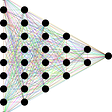## Genetic Algorithm Robot: Evolving Altitude, using Python, C++ and an Arduino.## The Ros Robot_localization package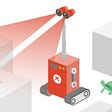## EXTRACTING PRIVATE DATA FROM A NEURAL NETWORK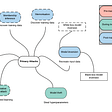## Dog Breed Classifier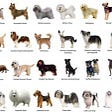## Towards the Democratization of Health: A Guide on Training and Deployment of Deep Learning Models…## [ Archived Post ] Random Notes for NLP Embedding## Bill Prin

Python, Data,Infrastructure, etc. https://billprin.com

## Google Cloud Platform: N-BEATS Component## Online prediction using GCP’s Vertex AI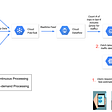## Using Google Cloud Machine Learning APIs programmatically in python - Part 2## A generalized end to end approach to regression problems using DNNs and Bigquery